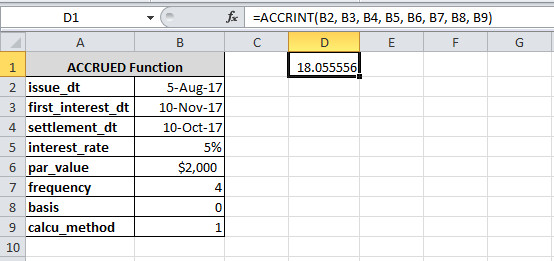# Excel ACCRINT Function To Return Accrued Interest

## What is ACCRINT Function in Excel

The ACCRINT function is the function of Microsoft Excel. It returns the collected interest for the security that pays interest on a periodical basis.

The function is categorized as Financial function in Excel.

## The Syntax of ACCRINT Function in Excel

The syntax given below contains the various parameters to pass. you have to enter only the required parameters to the function.

There are 8 parameters in the Excel ACCRINT function.

## Parameters or Arguments Passing to the Function

The description of the above parameters is given below. Check each parameter and enter only the valid value of these parameters.

Parameter NameDescription
issue_dtThe date when the security gets issued.
first_interest_dtThe date when the first interest of security will be paid.
settlement_dtIt is the settlement date which comes after the issue date.
interest_rateThe interest rate is the annual coupon rate for the given security.
par_valueIt is the par value of the given security. If you remove the par value, it assumes \$1000 as the default value of par value.
frequencyIt is the frequency of interest payment per year for the security. The frequency can be any value from payment like Annual(1), Semi-annaul(2), Quarterly(4).
[basis]The basis is the optional parameter of the function. It is the type of day count basis which is zero(0) by default. However, if it has a certain value then it can be any value from the below given.

ValueDay Count Type
0US (NASD) 30/360
1Actual/actual
2Actual/360
3Actual/365
4European 30/360
[calcu_method]It is an optional parameter. The parameter value is TRUE by default. However, it has a certain value from the given below value options.

ValueDescription
0When you calculate the interest from first_interest_dt to settlement_dt.
1If you calculate the interest from issue_dt to settlement_dt.

## Example To Get the Accrued Interest for the Security

To get the accrues interest for the security, you have to follow the example given below.The date format for the parameter should be taken from the cell of an excel sheet. You can use the above example by entering the parameter values in the cell. After that, you have to put the cell name with the parameters.

One more thing, you have to notice here that you cannot put the above data formats directly to the function. Read further to get the valid formats to put directly.

Put parameter values directly to the function

To put values to the function directly, you have to use the below-given example. The dates cannot be put directly to the function. You have to use the `DATE()` to put the dates and get the output.

Also, you have to use the percentage(%) sign for the rate. Without the sign, you will get the output multiplied by 100.

Output

18.05555556

In the above example, you can also use 0.05 for the rate in place of 5%. The output given above gives accrued interest for the security,

Hope, you like this post of how to use ACCRINT function to return accrued interest. If you have any query regarding the tutorial, please comment below.

Also tell me, what other uses you are performing with the function.